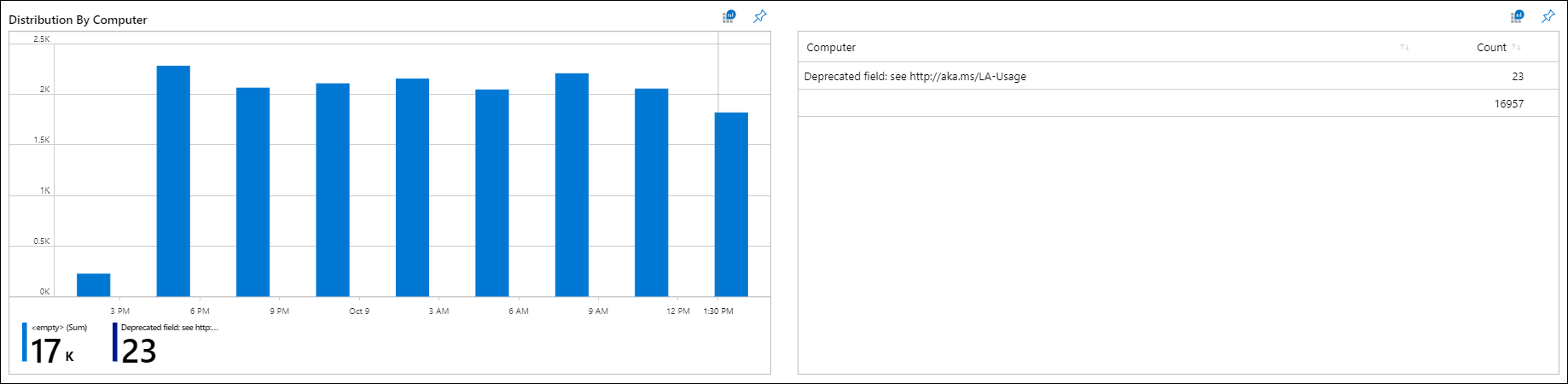# Azure Monitor 视图设计器磁贴转换Azure Monitor view designer tile conversions

## 圆环图和列表磁贴Donut & list tile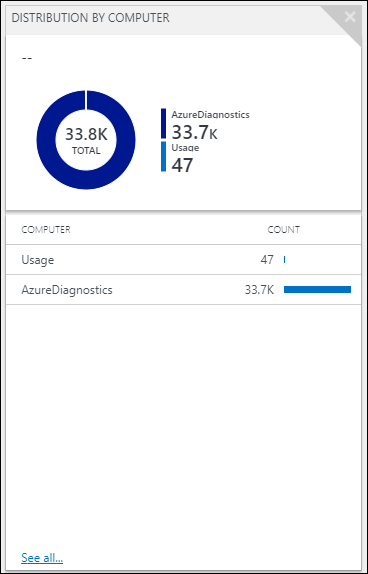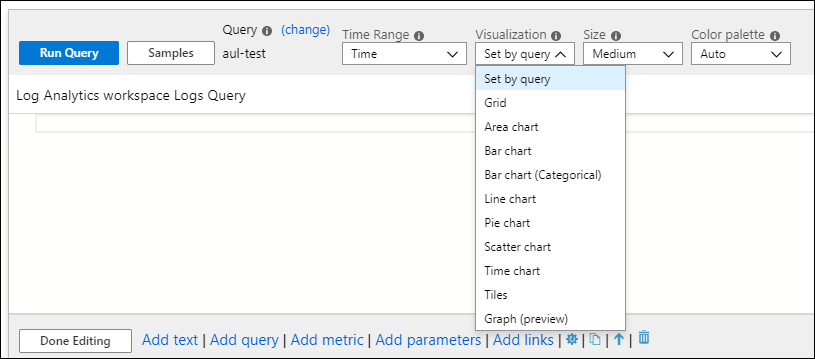``````search *
| summarize AggregatedValue = count() by Type
| order by AggregatedValue desc
``````

``````search *
| summarize AggregatedValue = count() by Type
| order by AggregatedValue desc
| render piechart
``````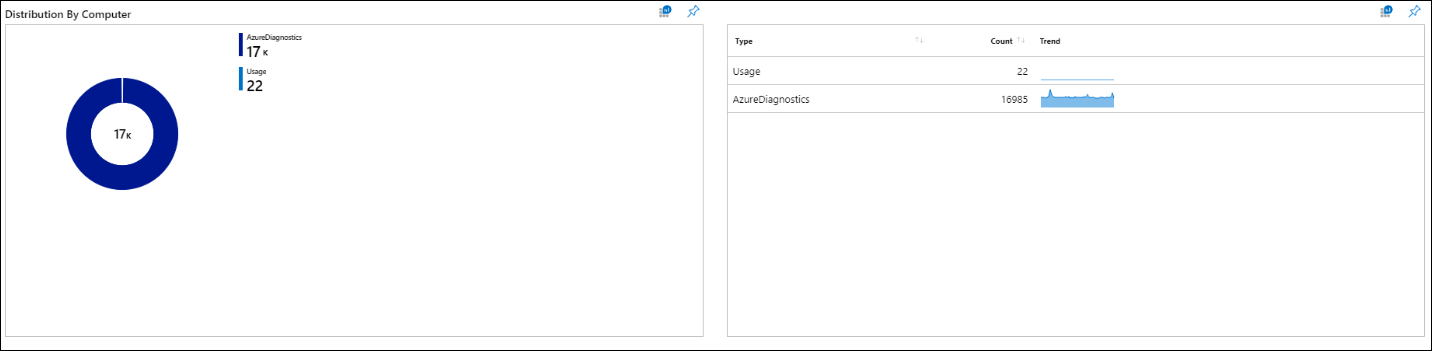## 折线图和列表磁贴Line chart & list tile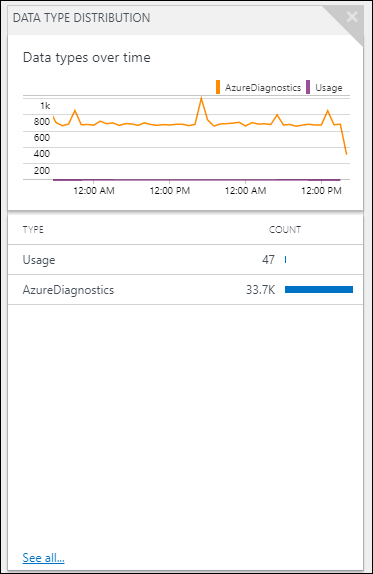``````search *
| summarize AggregatedValue = count() by Type
``````

``````search *
| make-series Count = count() default=0 on TimeGenerated from {TimeRange:start} to {TimeRange:end} step {TimeRange:grain} by Type
``````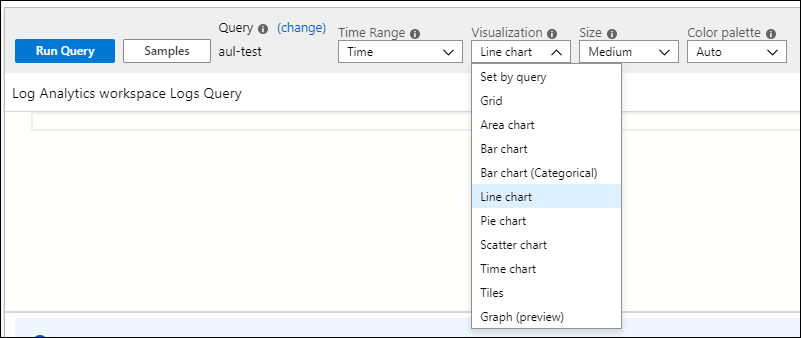``````search *
| make-series Count = count() default=0 on TimeGenerated from {TimeRange:start} to {TimeRange:end} step {TimeRange:grain} by Type
| render linechart_
``````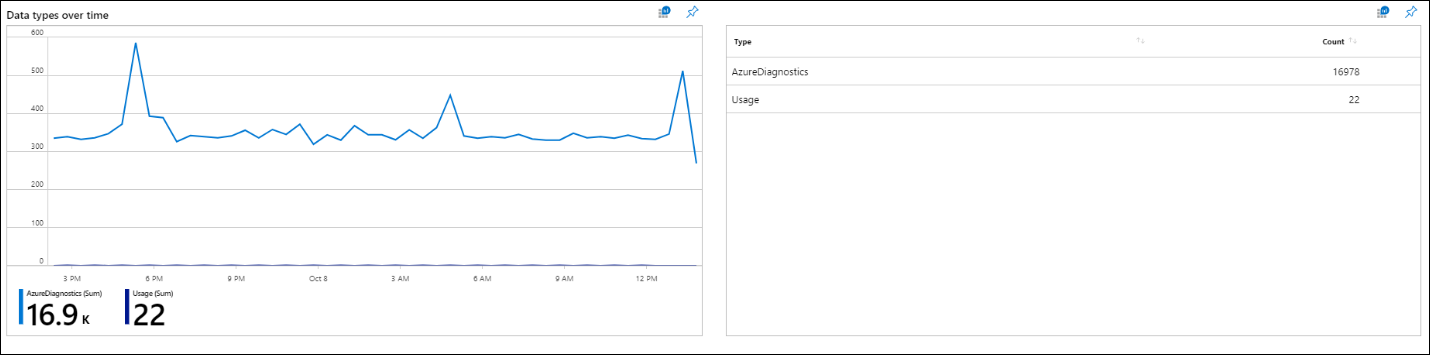## 数字和列表磁贴Number & list tile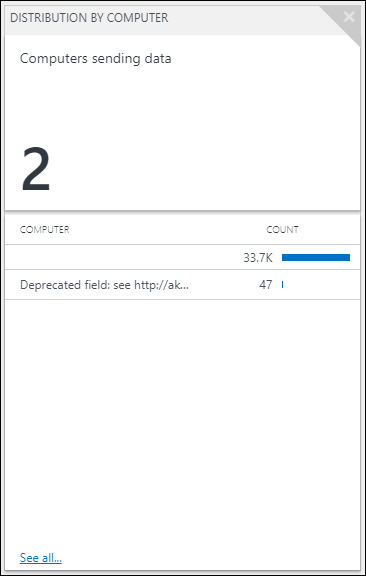``````search *
| summarize AggregatedValue = count() by Computer | count
``````

``````search *
| summarize AggregatedValue = count() by Computer
| summarize Count = count()
``````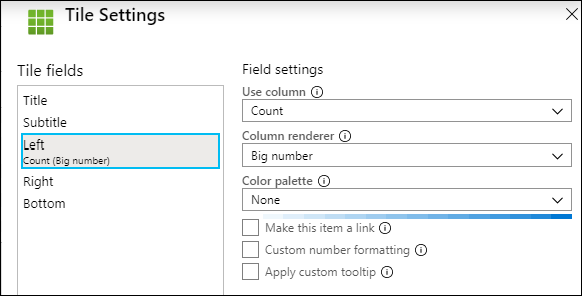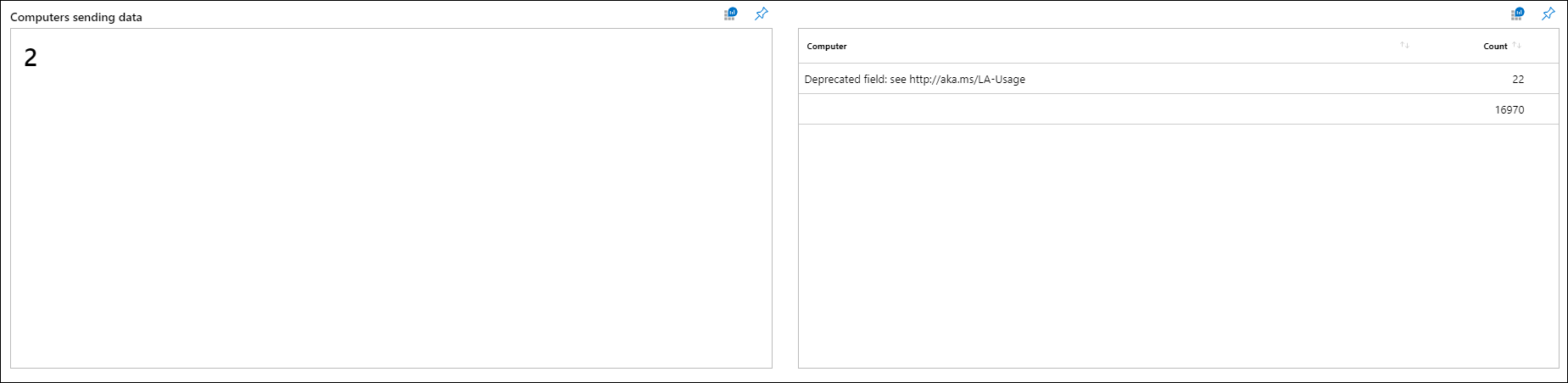## 时间线和列表Timeline & List``````search *
| sort by TimeGenerated desc
``````

``````search *
| summarize Count = count() by Computer, bin(TimeGenerated,{TimeRange:grain})
``````